# NCERT Solutions for Class 10 Maths Chapter 14-Statistics

NCERT Solutions for Class 10 Maths Chapter 14-Statistics

Class 10 Maths NCERT solutions, Chapter 14 Statistical. Solutions are prepared by our math experts. All solved statistics questions are the latest updates to the CBSE syllabus and guidelines to help students solve each practice question and prepare for the CBSE 2023 exam. Using these solutions as reference tools can help students achieve good grades. Students can also get answers and practice problem solving through exercises in each of the 10 math chapters.

NCERT Solutions for Class 10 Maths Chapter 14-Statistics | Free PDF Download

The topics included in Chapter 14, Statistics are:

• Mean of Grouped Data
• Mode of Grouped Data
• Median of Grouped Data
• Graphical Representation of Cumulative Frequency Distribution

NCERT solutions Class 10 Maths Chapter 14 - Statistics is available for students who want to perform well in this chapter. Problem-solving methods and procedures are clearly explained in these NCERT solutions so that students can quickly grasp the basics.

The world is strongly data-driven, and virtually every domain has a data-oriented group that represents relevant information. Statistics is a branch of mathematics that deals with the representation of data in a meaningful way.

You will encounter many real-life scenarios in which you use statistical basics to display a series of data in the form of tables, graphs, or pie charts. In this chapter, you will learn methods such as the stepwise deviation method, finding the mode and median of grouped data, transforming frequency distributions, the relationship between the mean and the median, and mode. Class 10 NCERT solutions are the best research. Materials to prepare for the CBSE 2023 exam.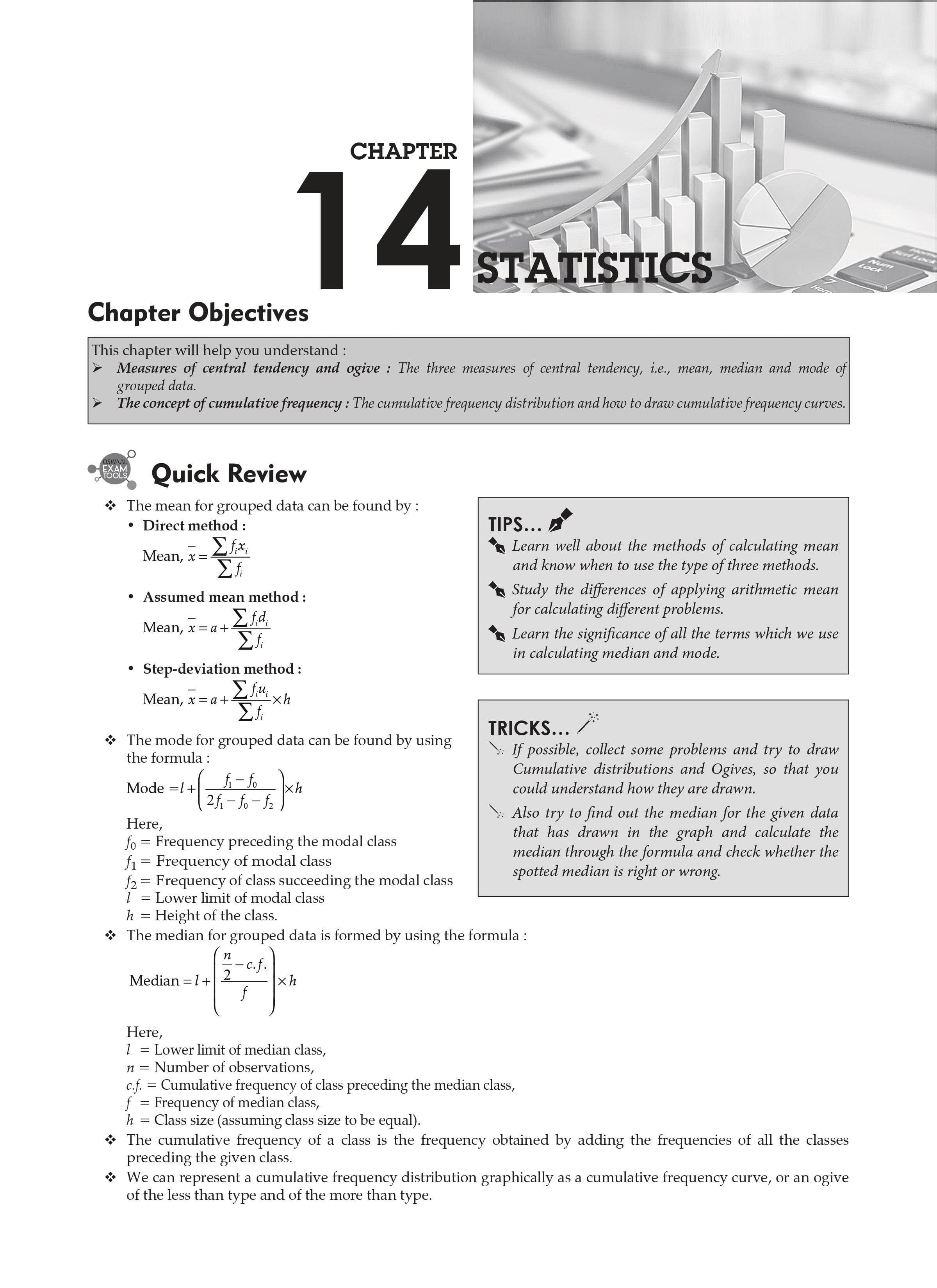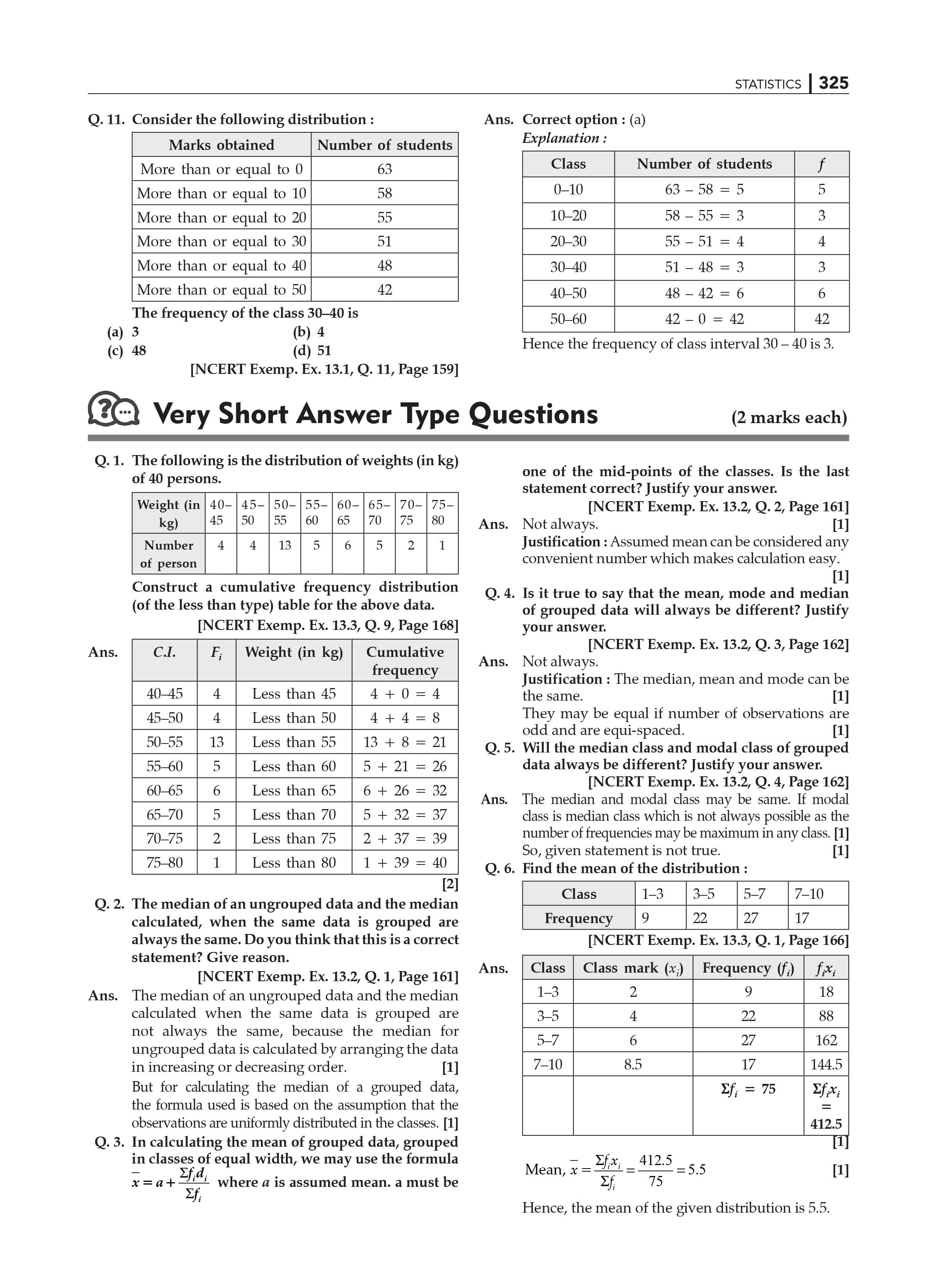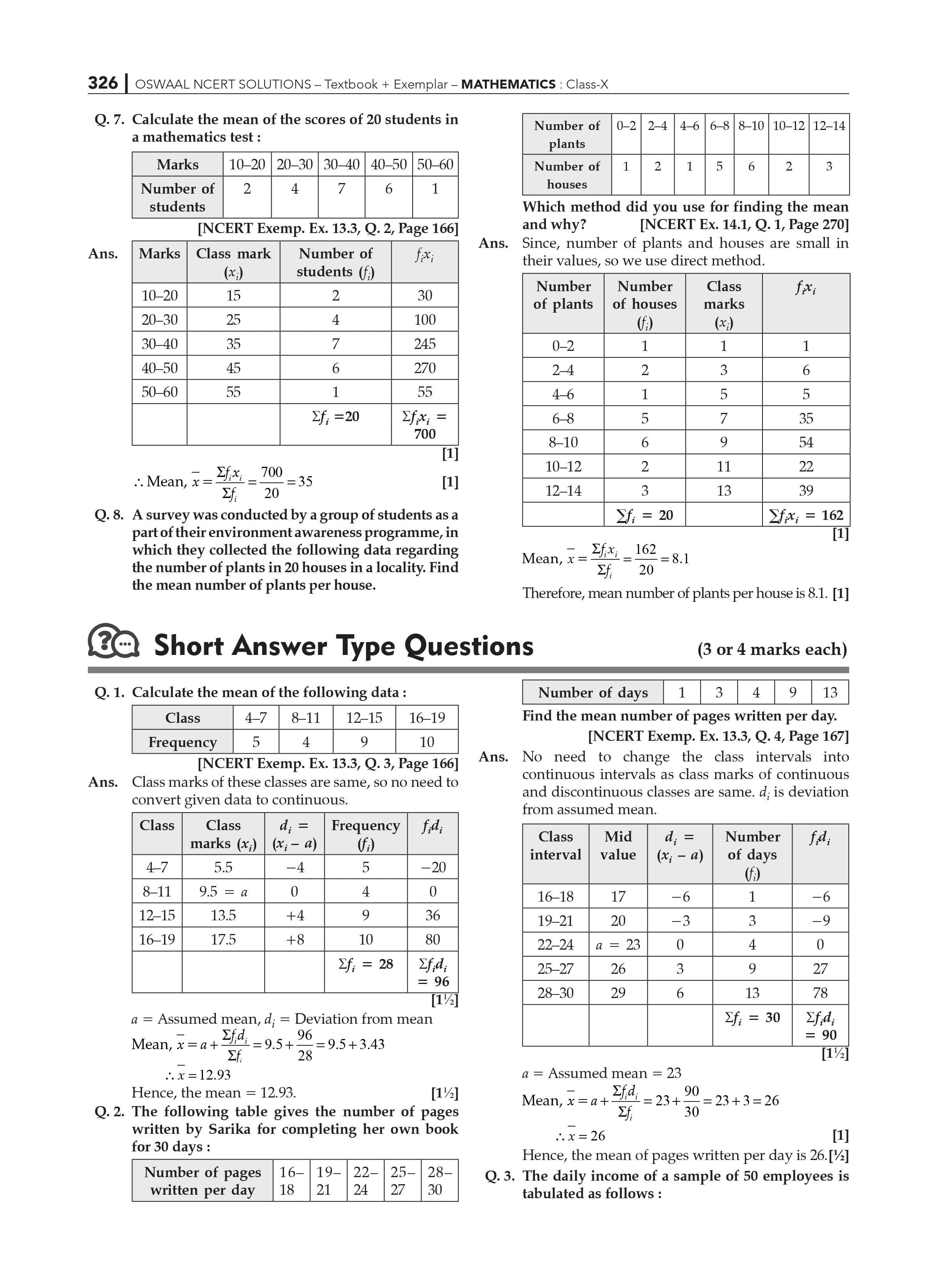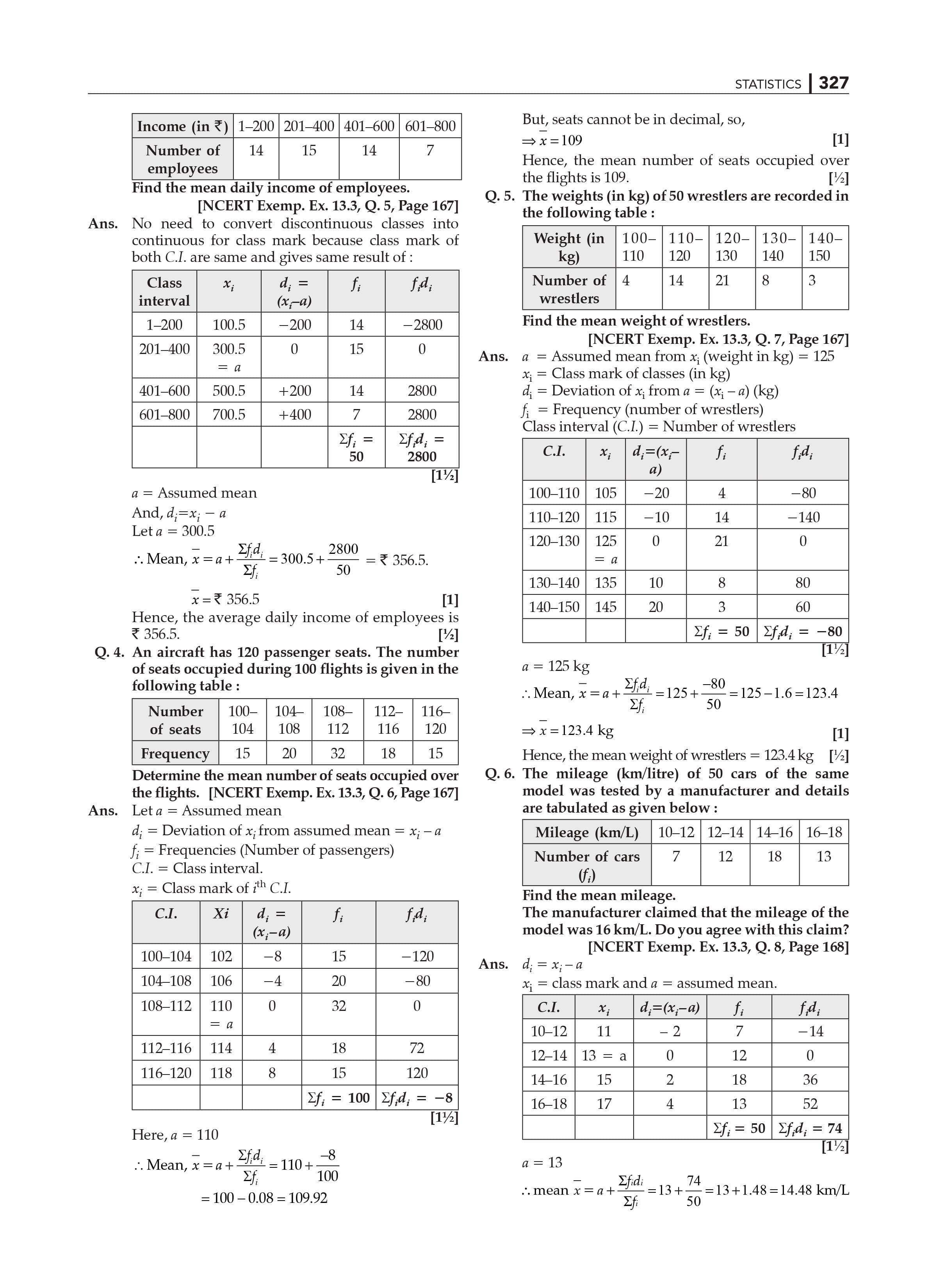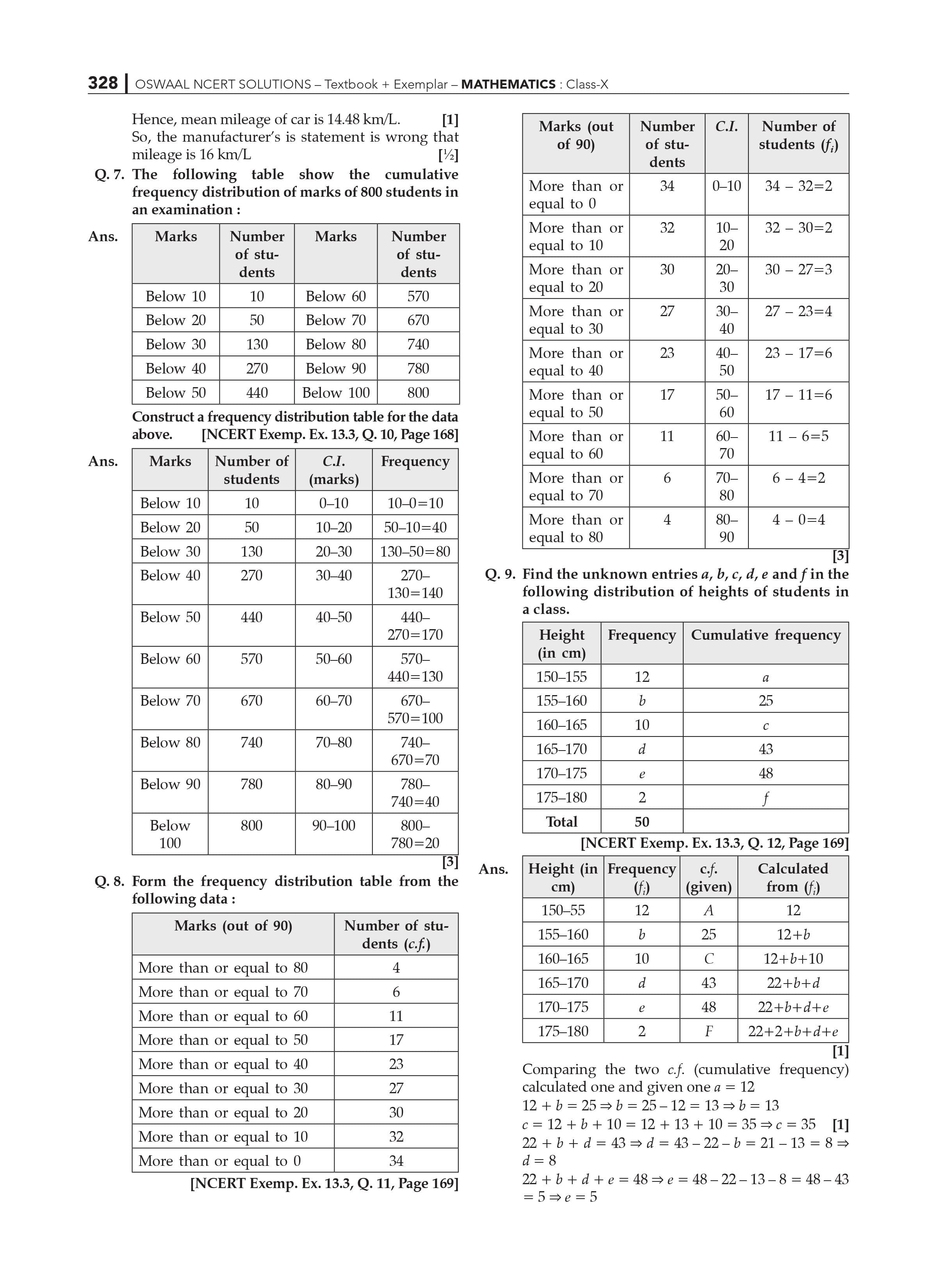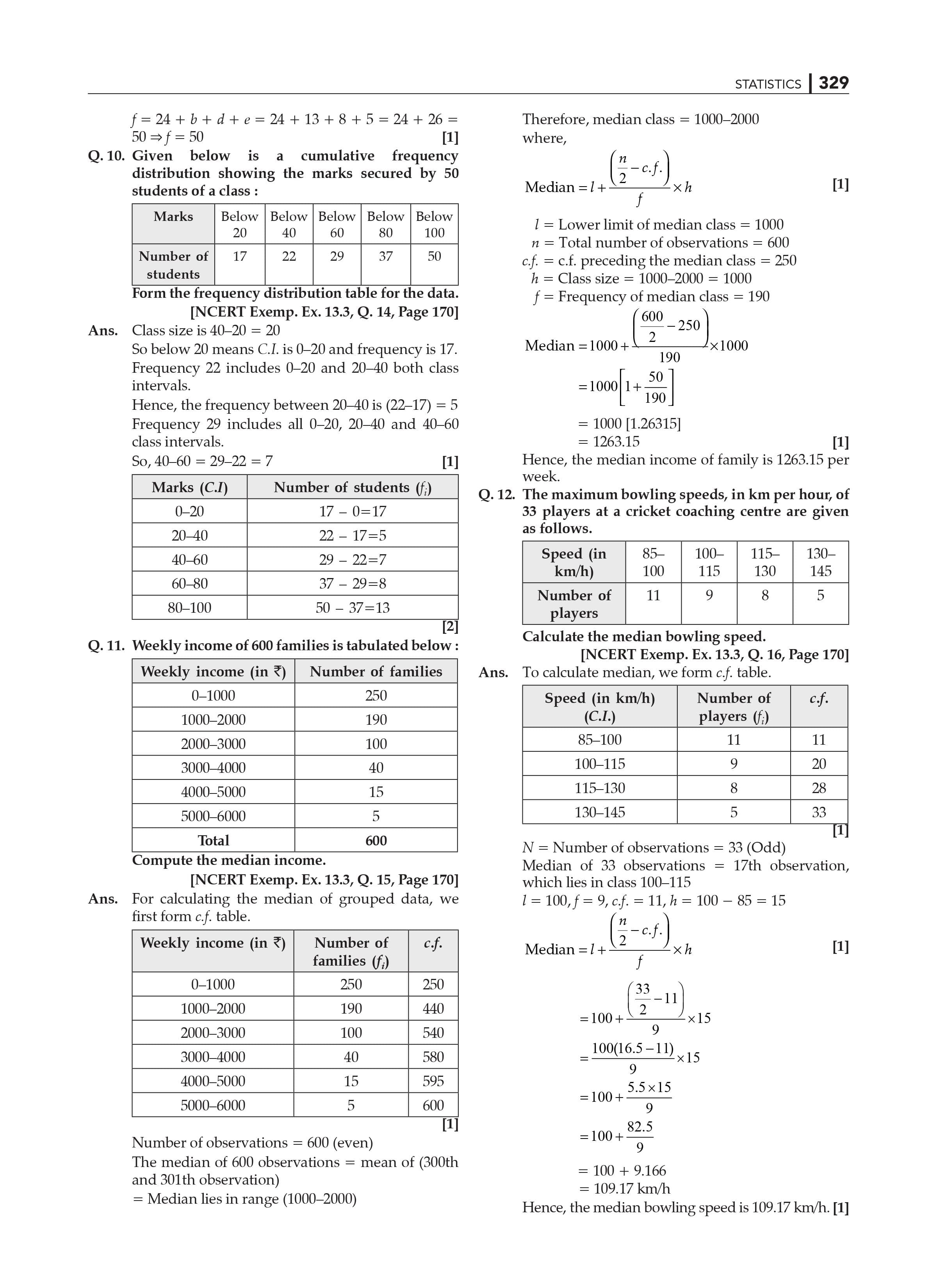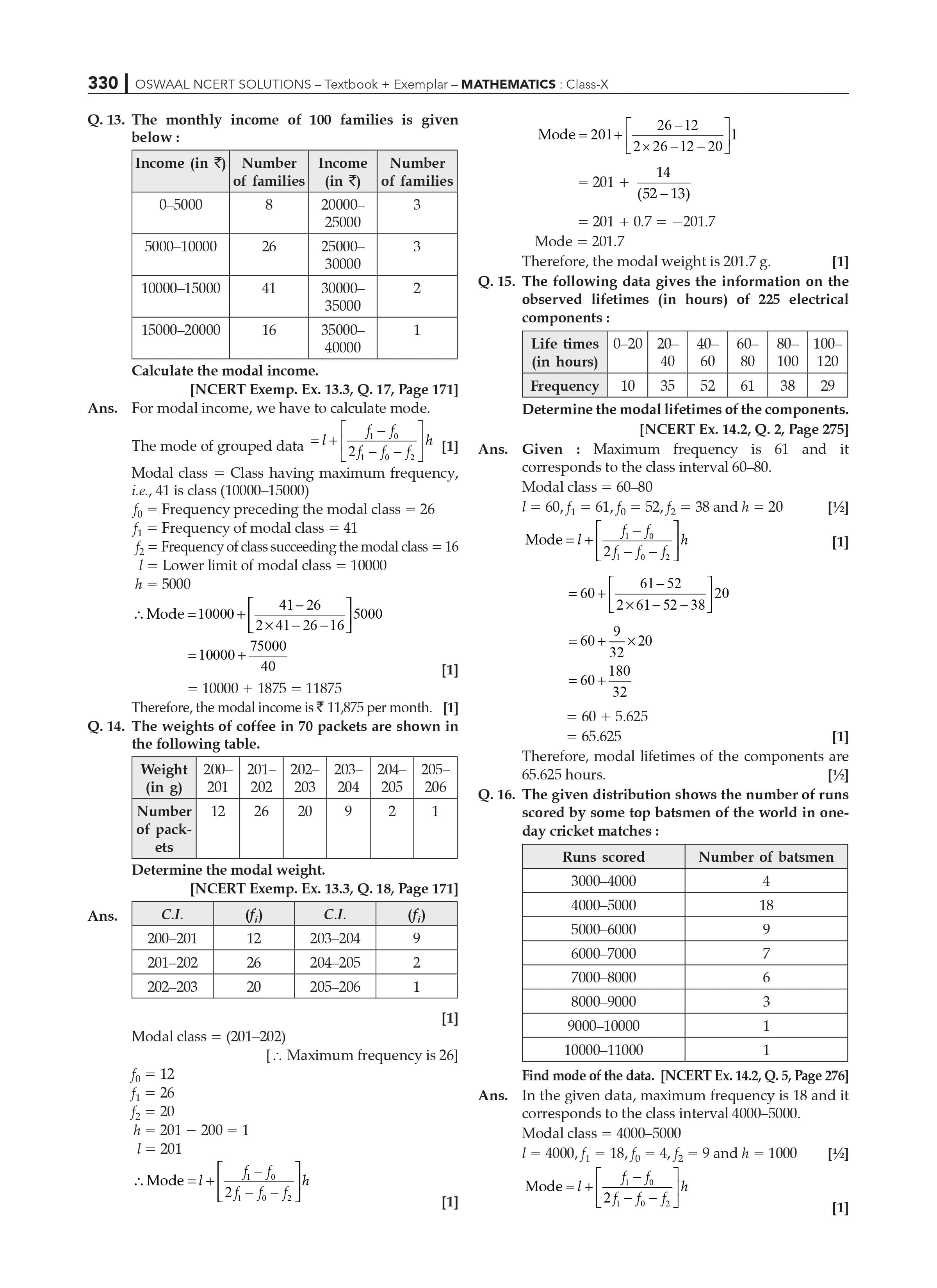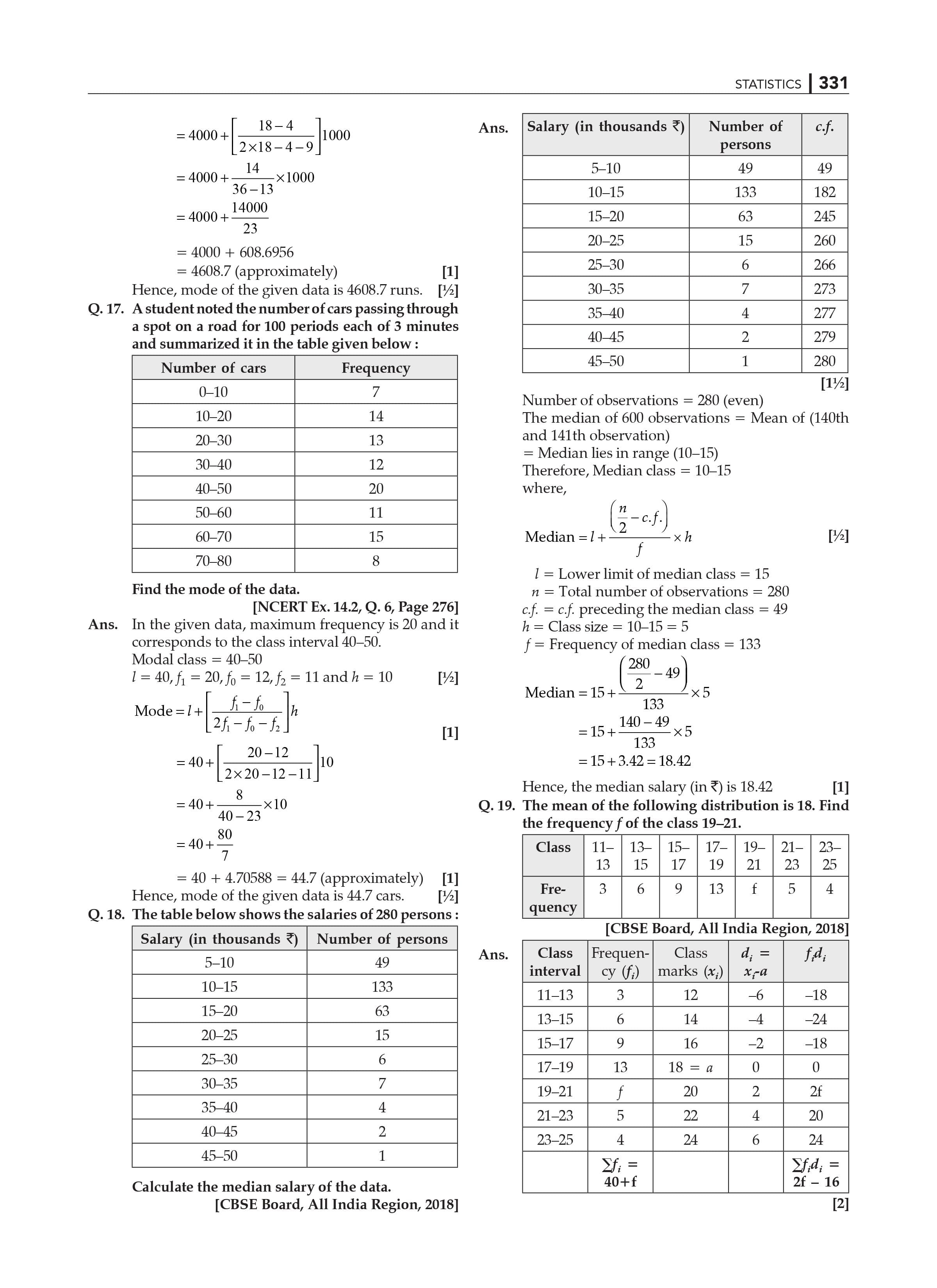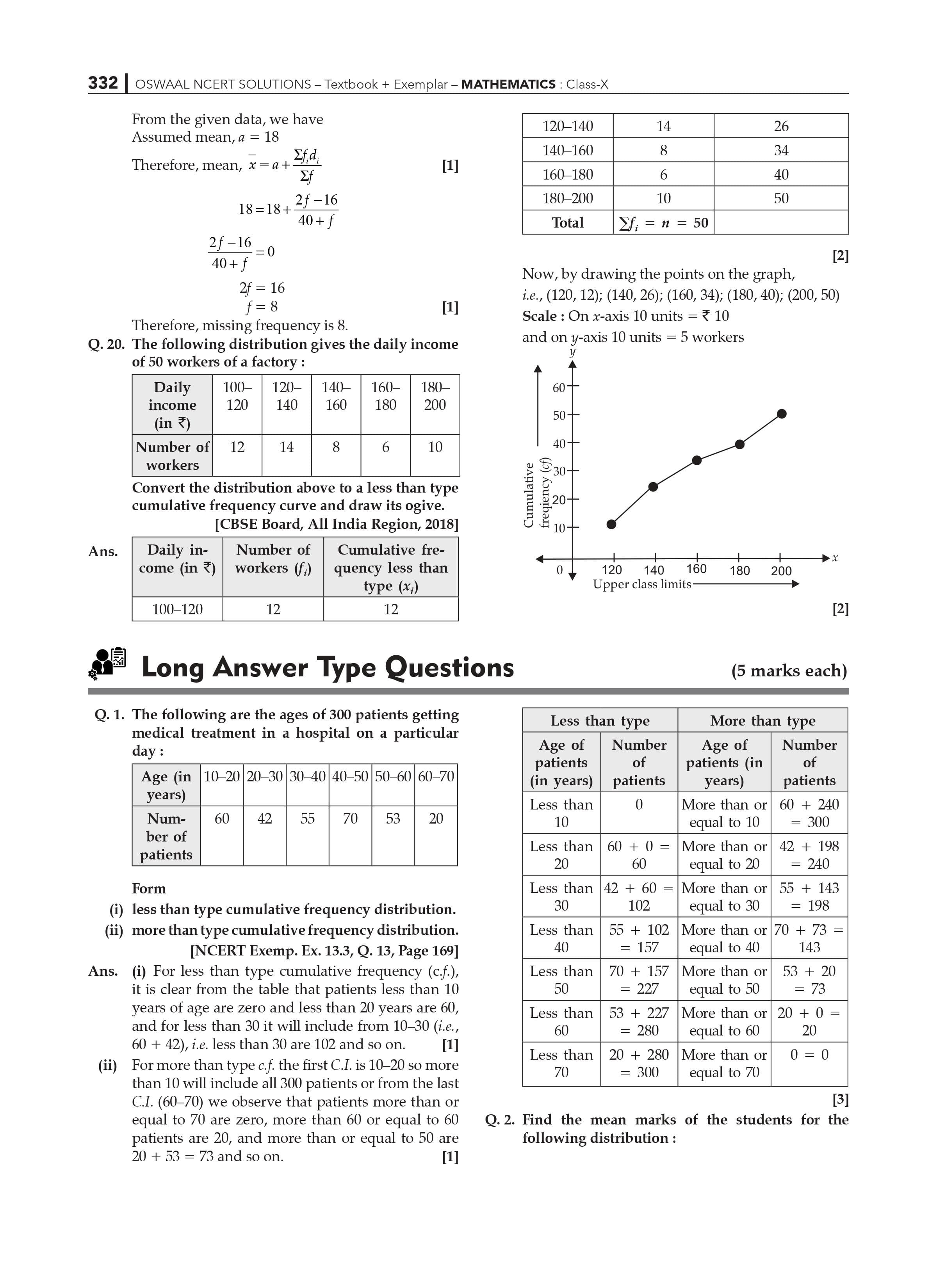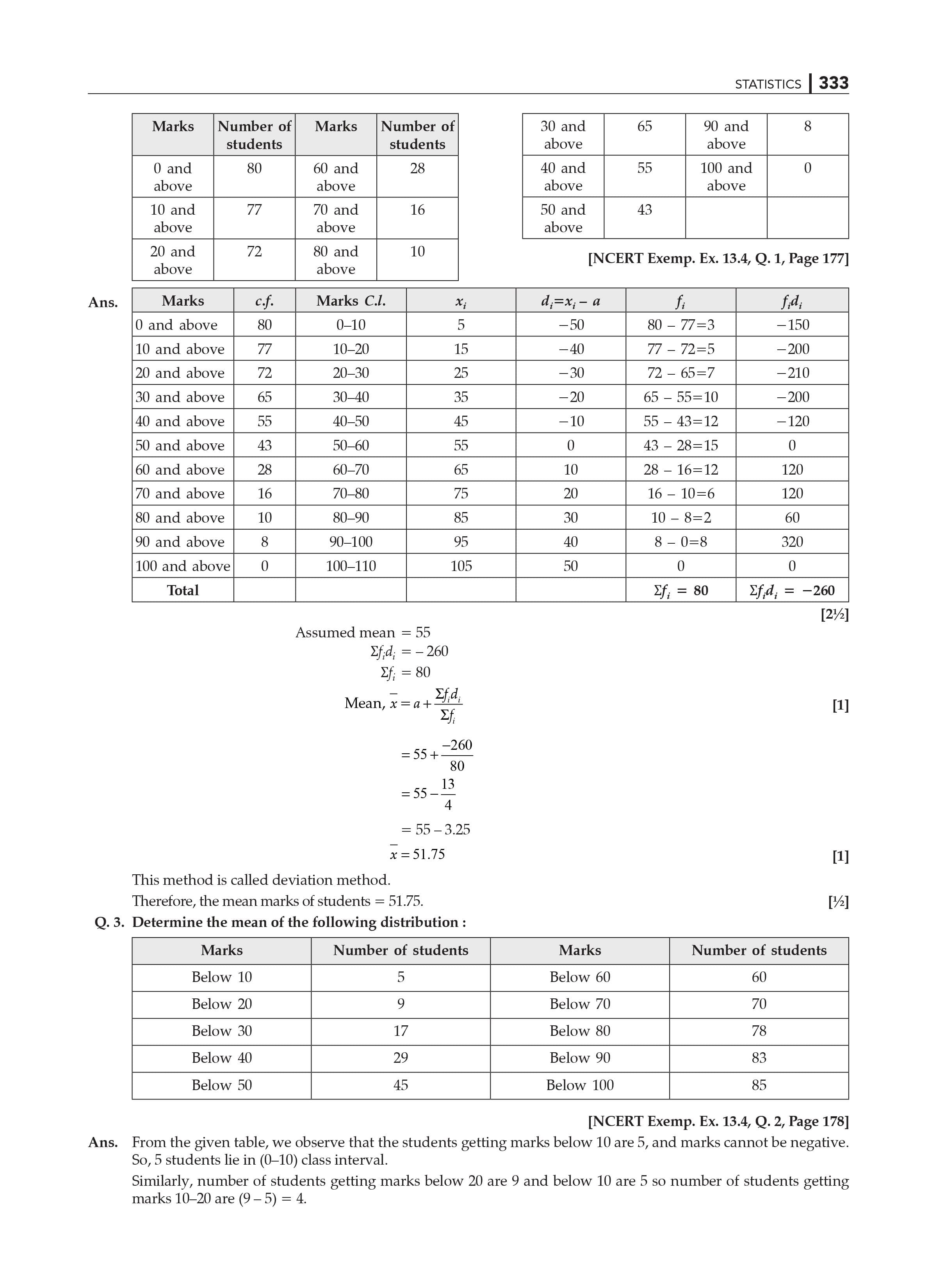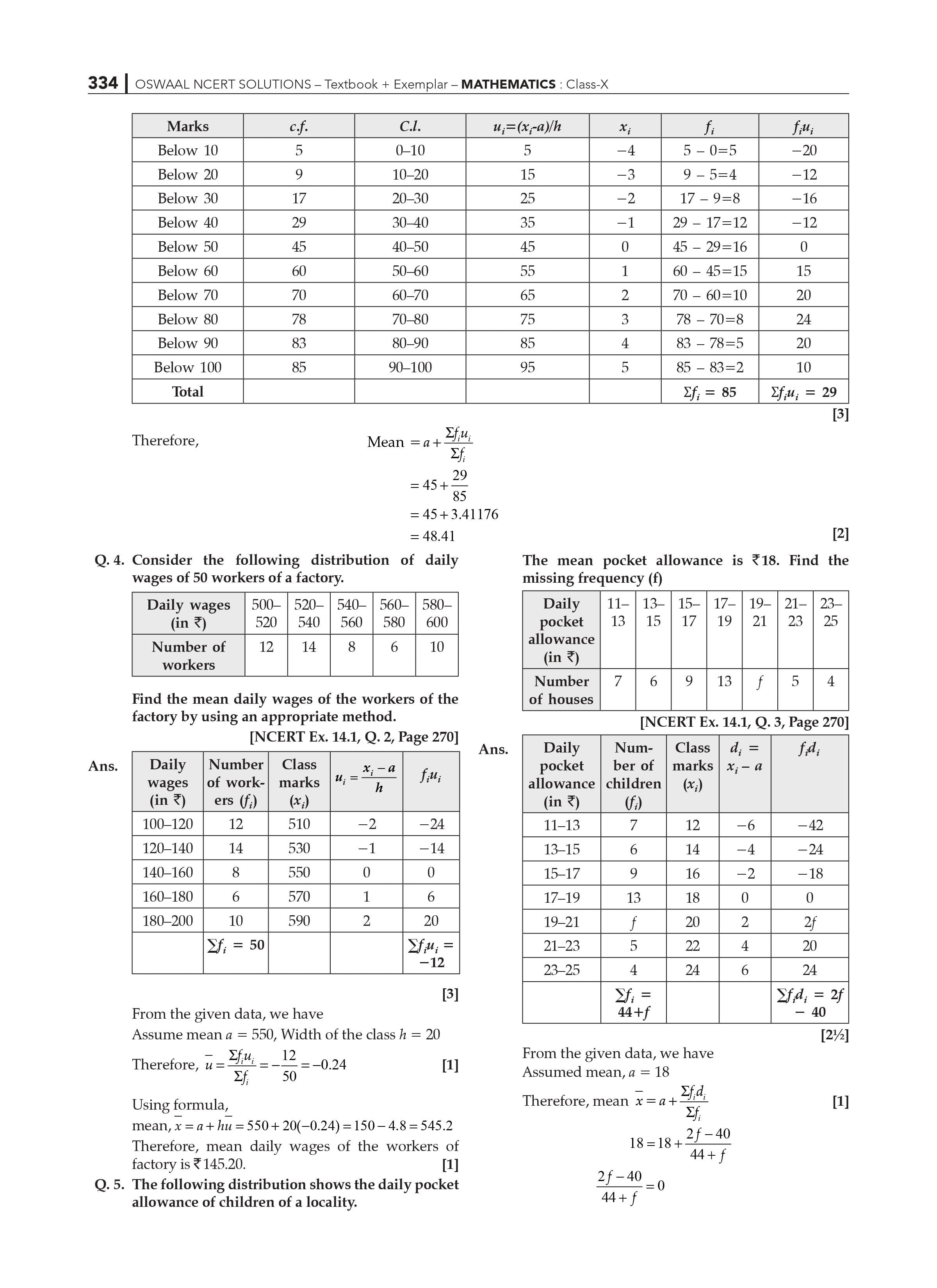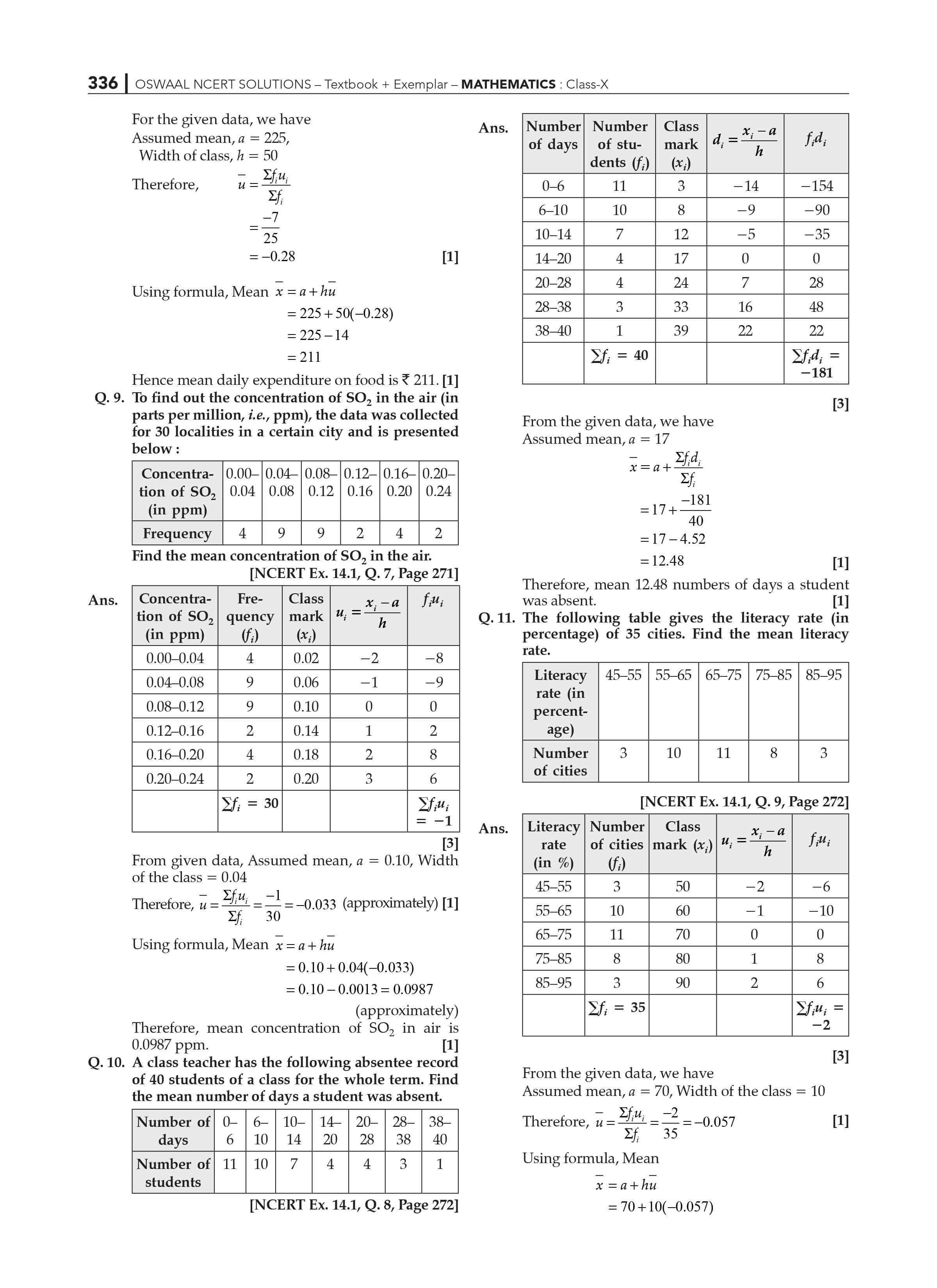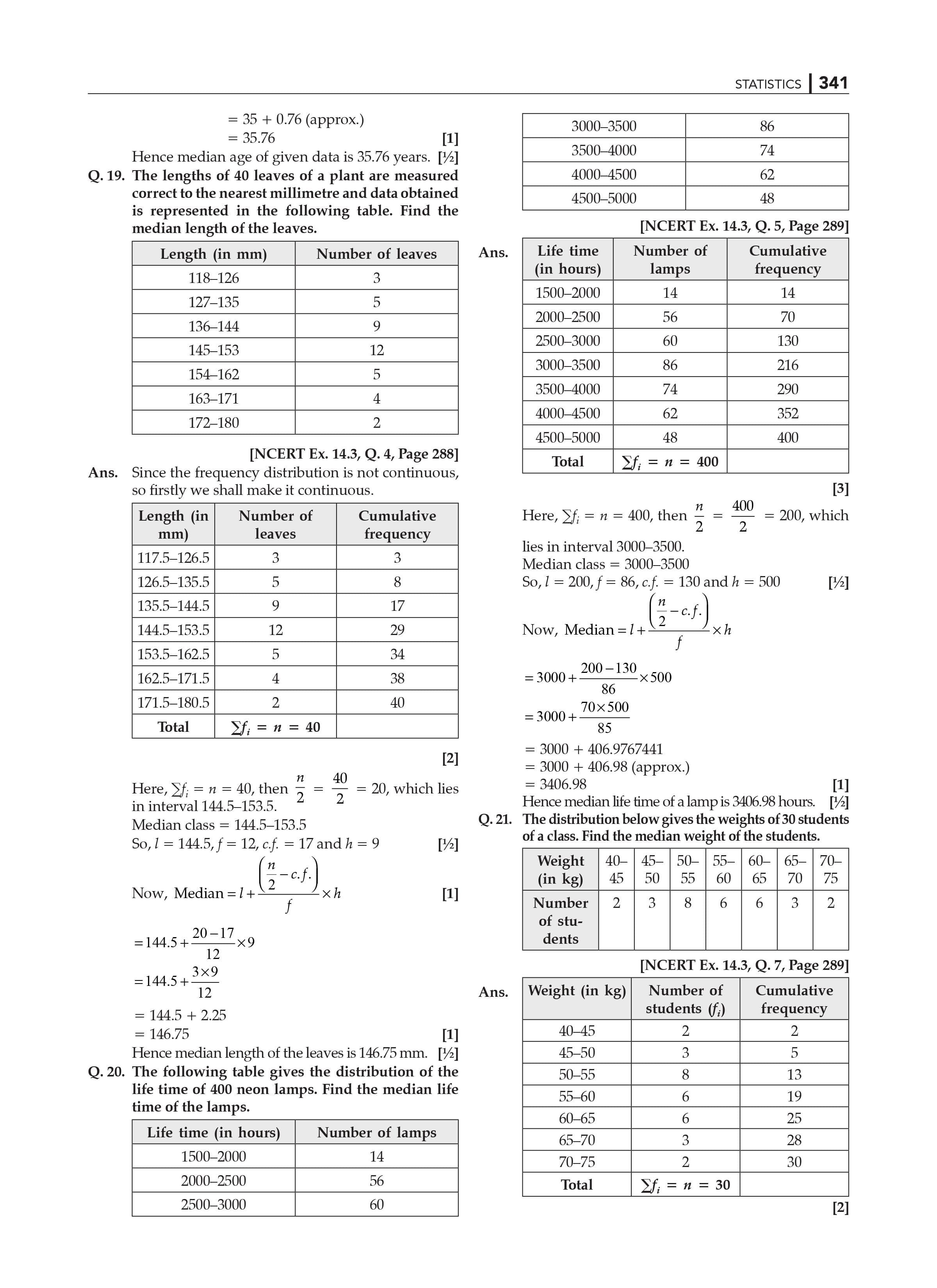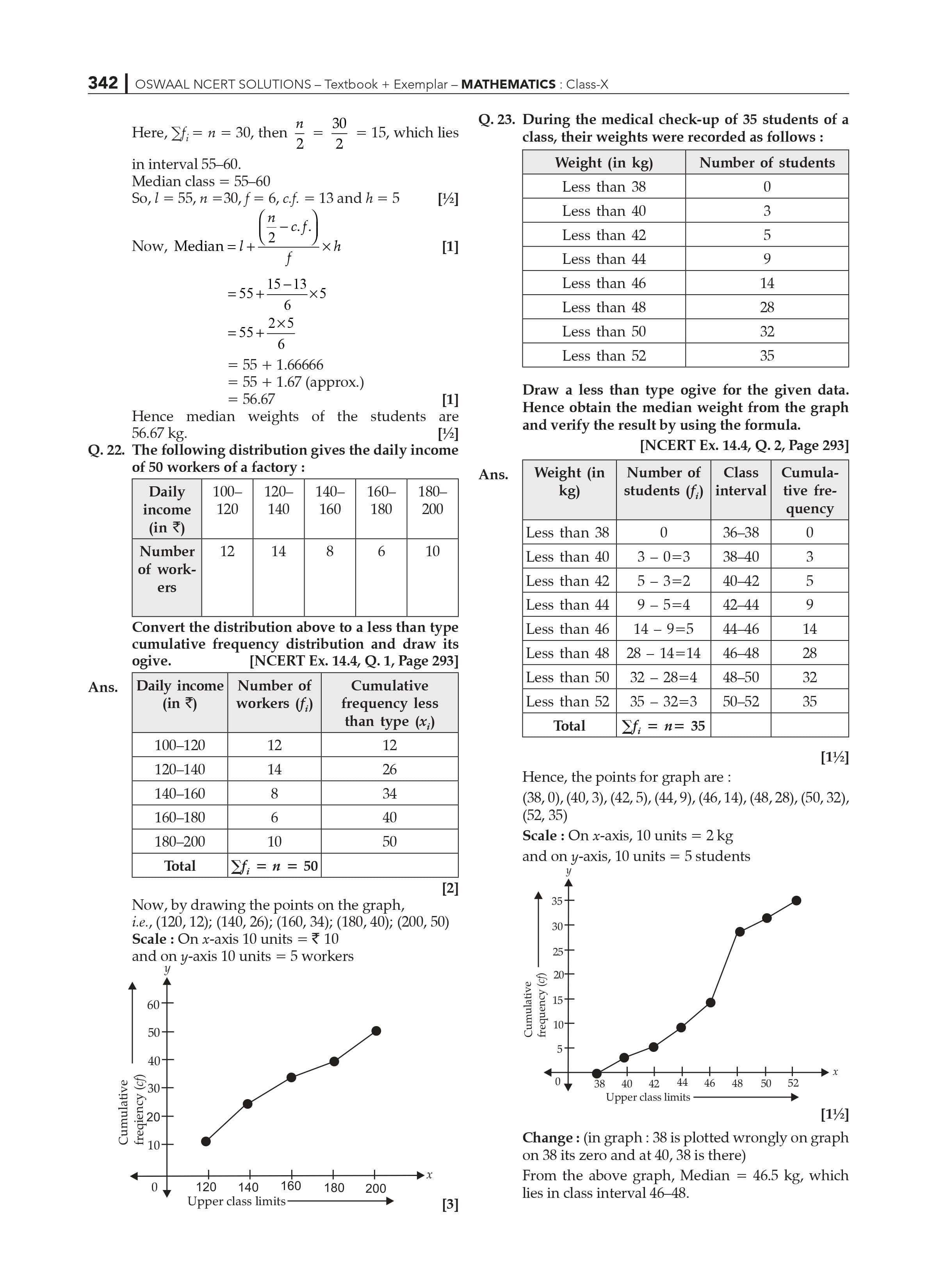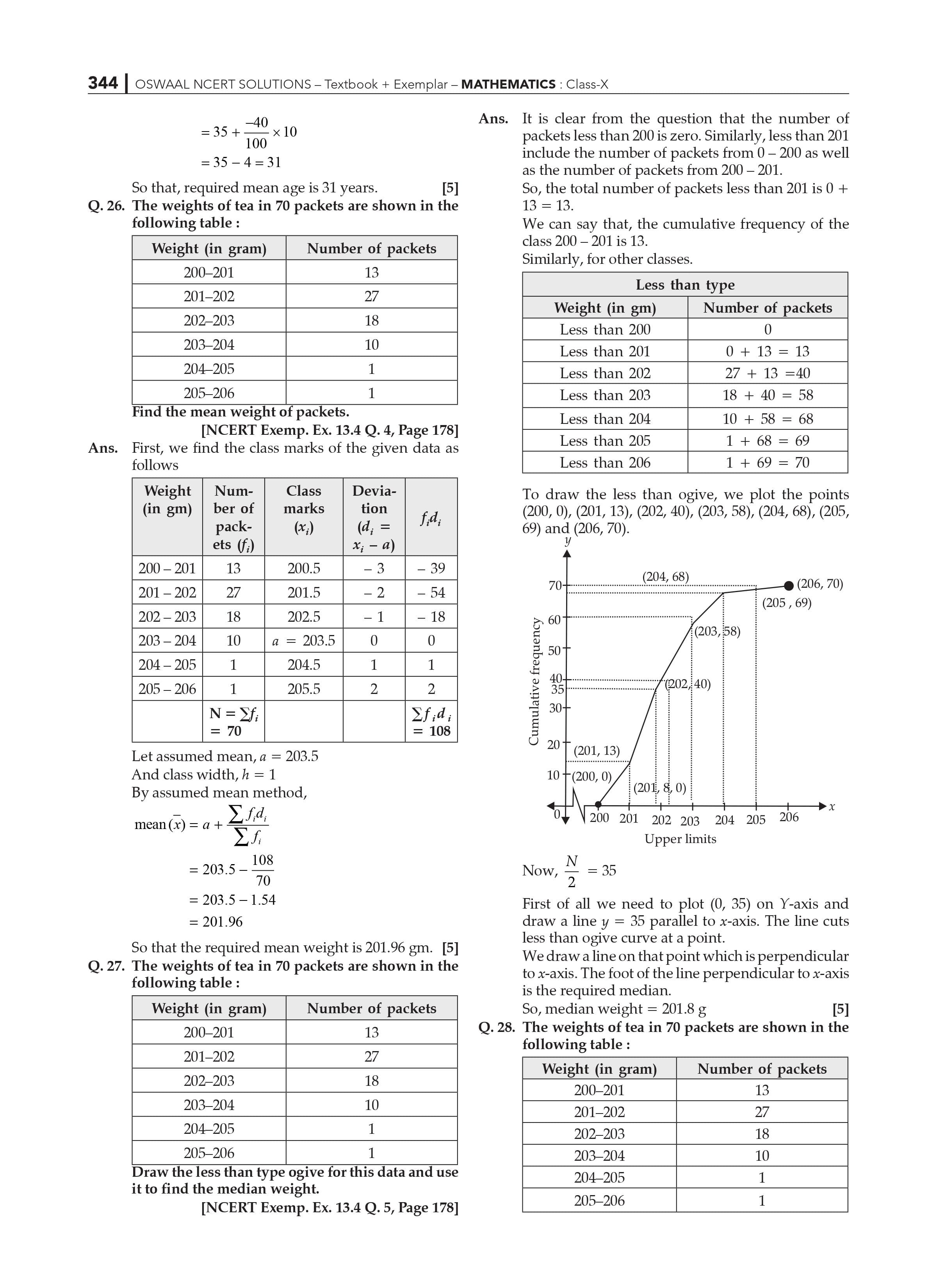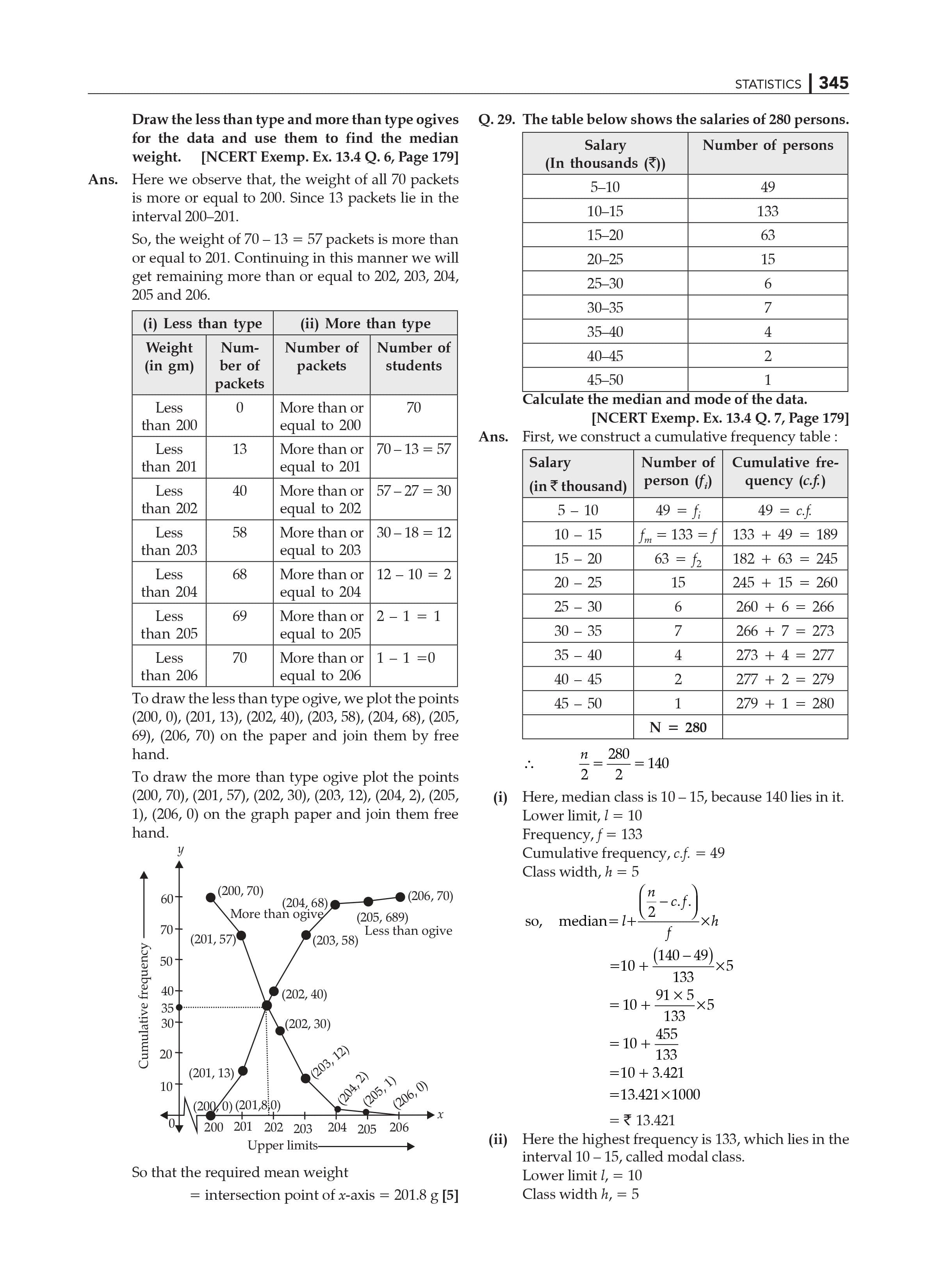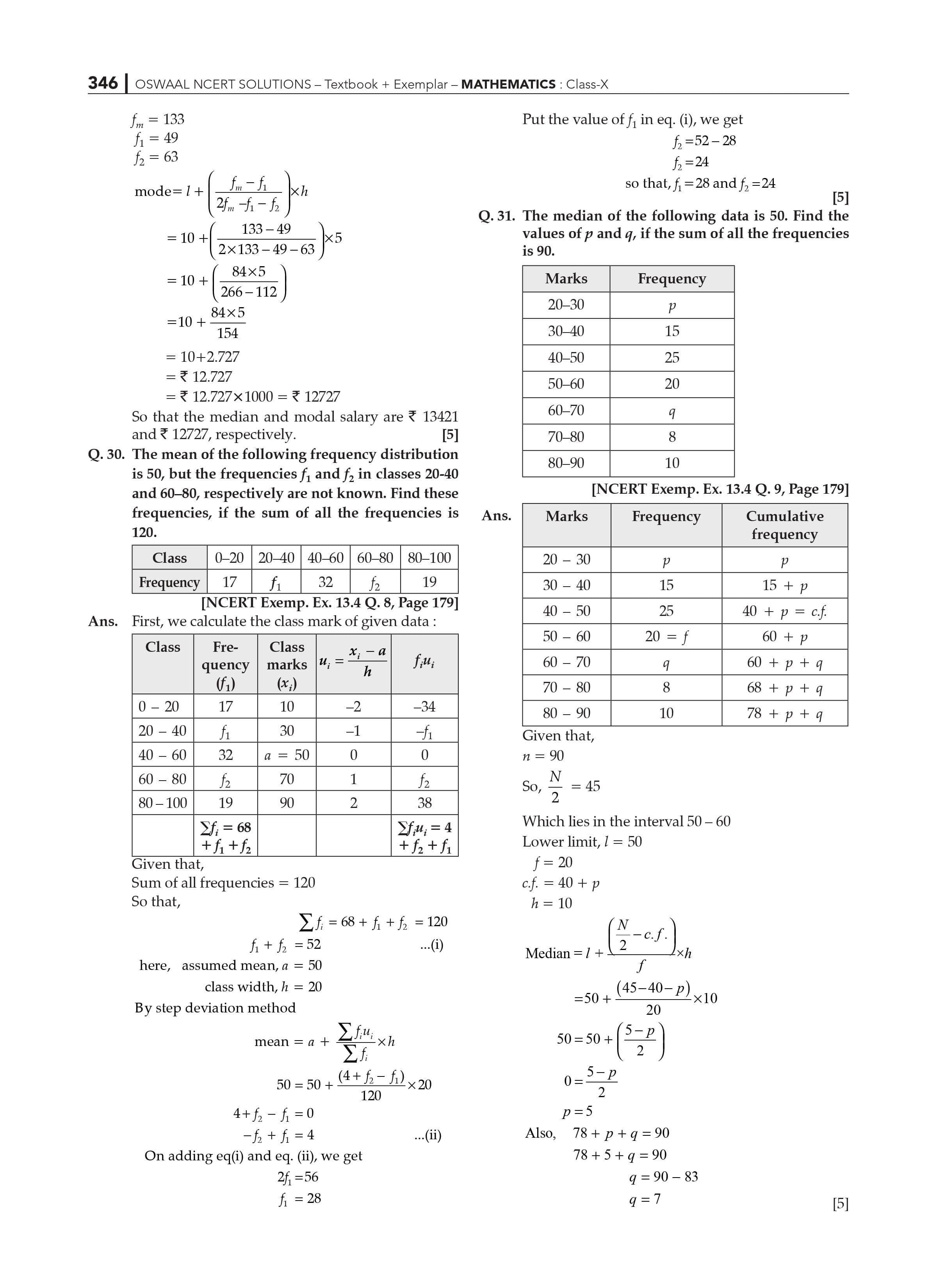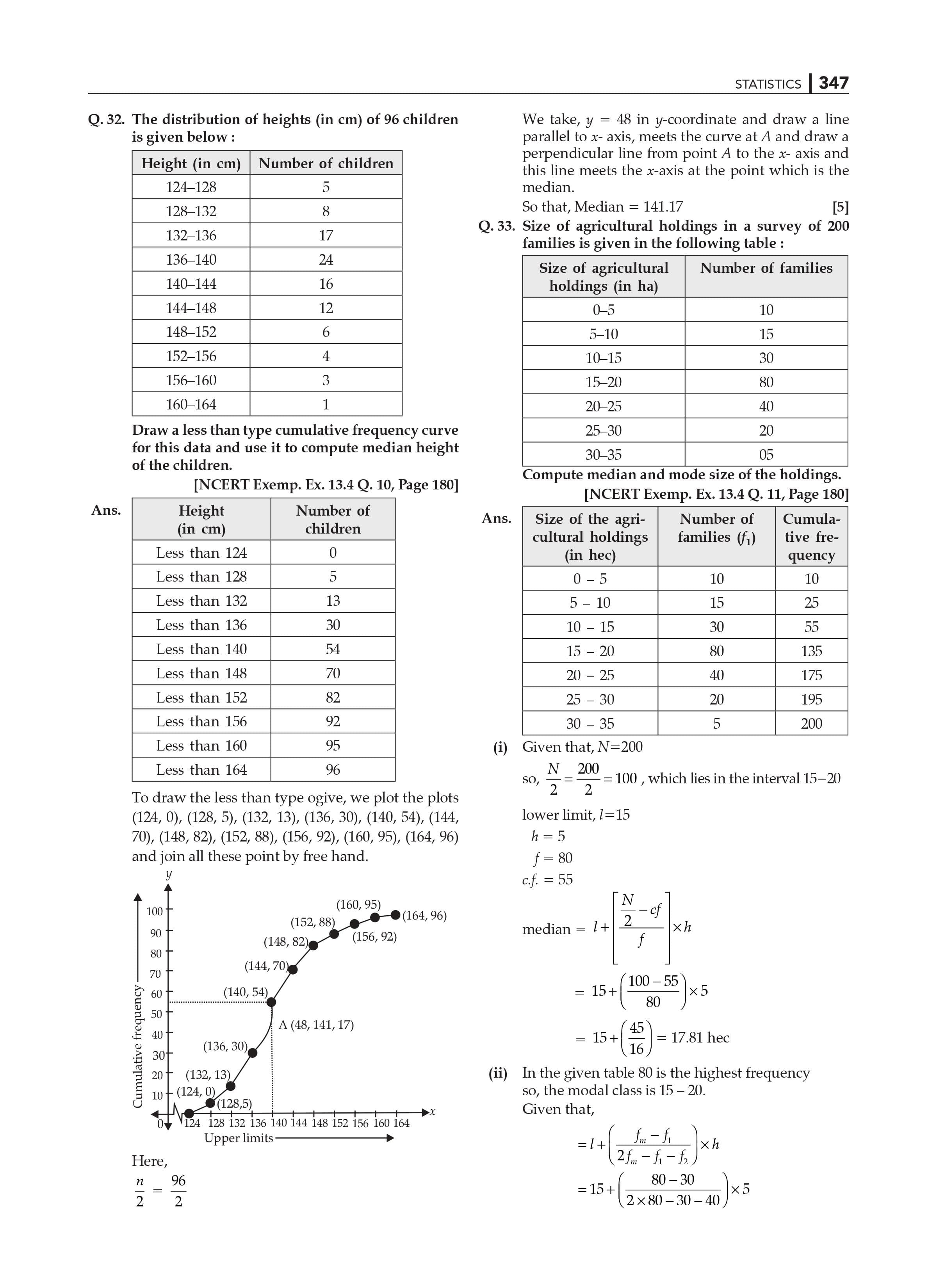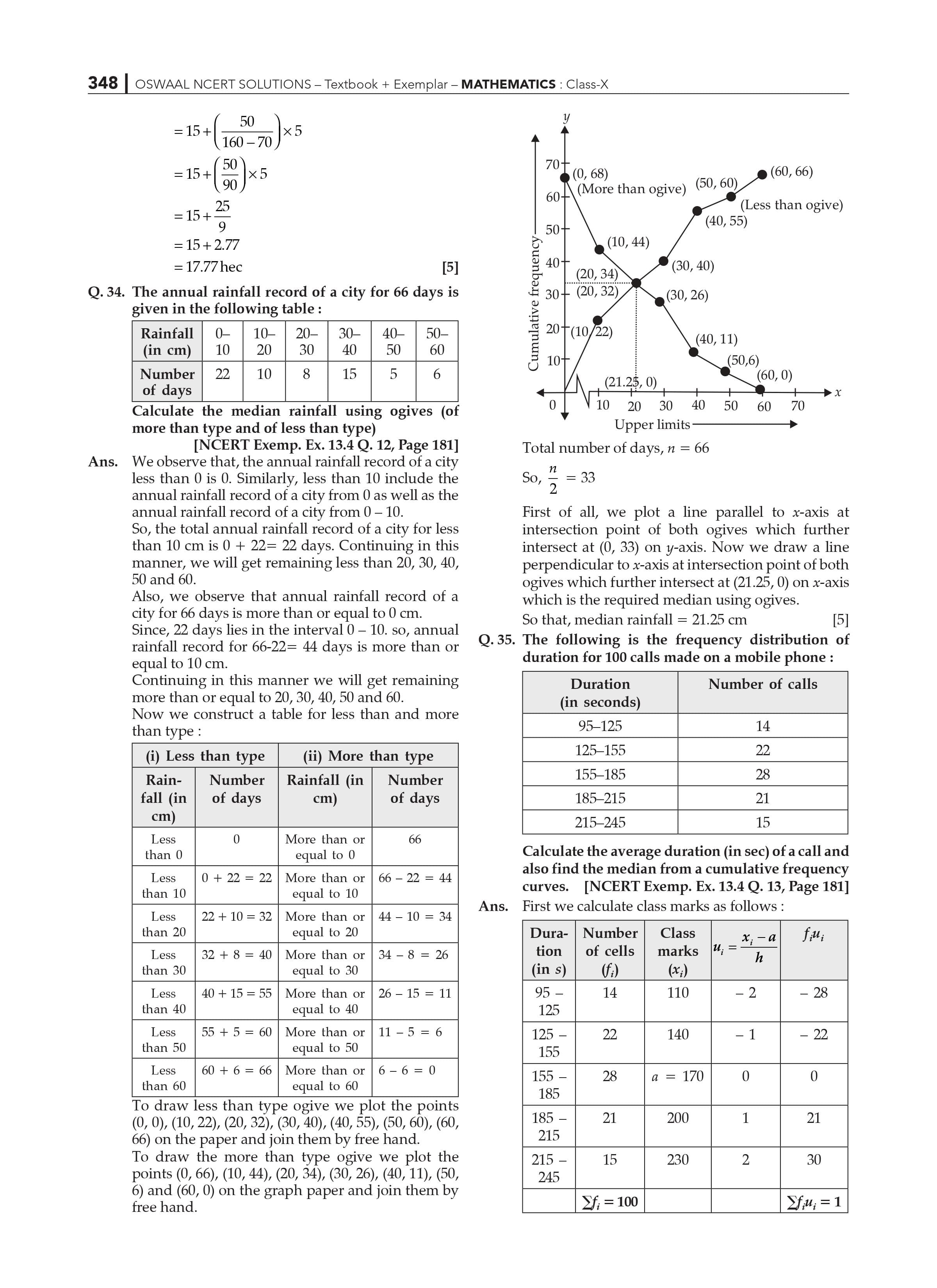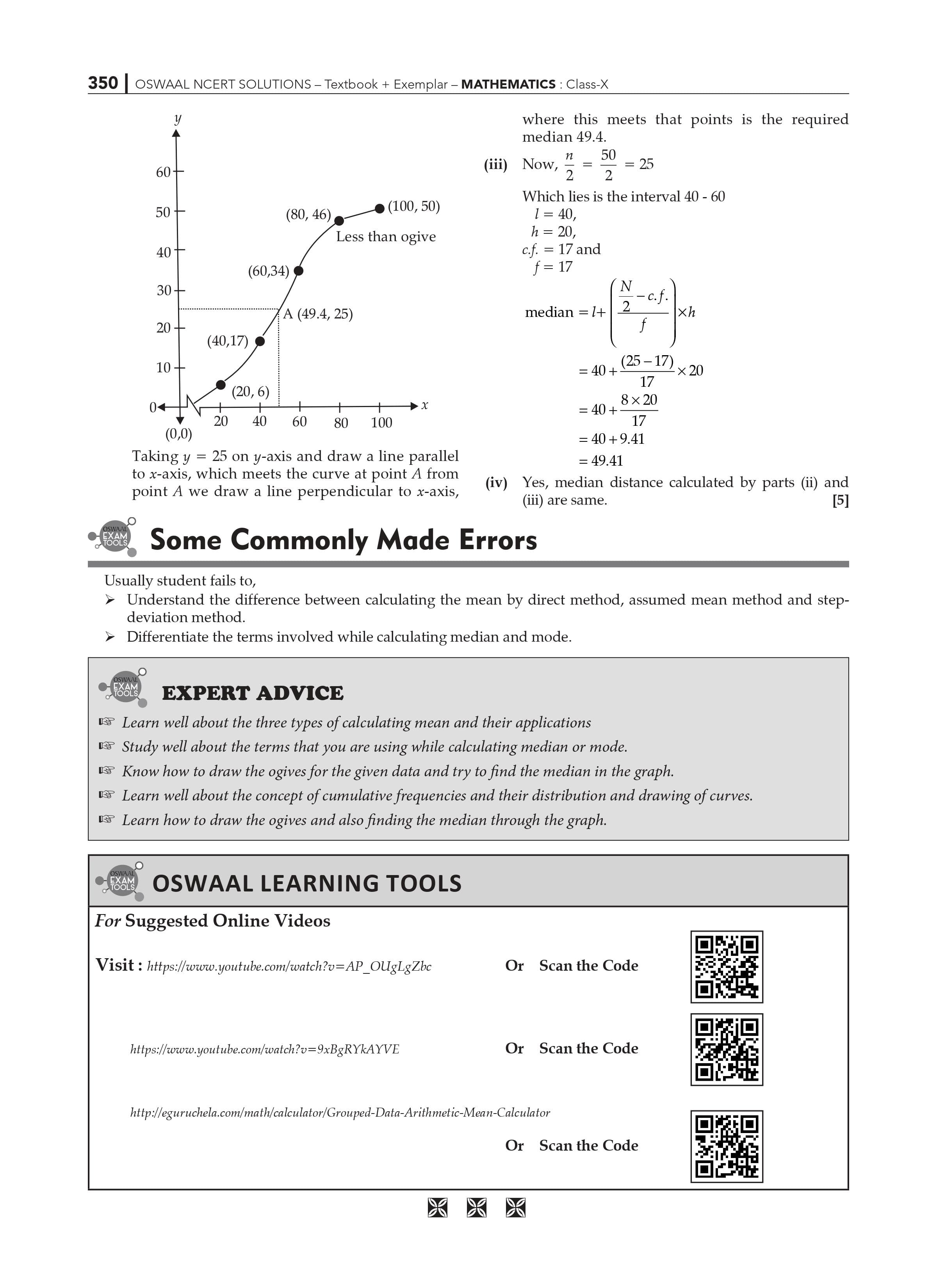Key Features of the NCERT solutions class 10 Maths Chapter 14 - Statistics

The solutions in the statistics chapter are for students' reference.

• In this way, students can score well in this statistics chapter.
• With this resource, students can prepare and can do multiple revisions for this Chapter 14.
• Statistics questions are answered by subject matter experts.
• The content of the material is as per the CBSE syllabus (2022-2023) and guidelines.
• Statistics can also be better understood using NCERT solutions. These solutions are designed to help students do well on CBSE exams.

 Extra Information: NCERT Exemplar for Class 10 Maths Chapter 14 CBSE Notes for Class 10 Maths Chapter 14

Frequently Asked Questions for CBSE Exams, Solutions for NCERT Class 10 Maths Chapter 14:

1. Is the NCERT solution for class 10 Chapter 14 Maths help you pass the CBSE Term 2 exam?

Yes, Chapter 14 of NCERT Solutions Class 10 Maths is one of the important chapters of NCERT Solutions Class 10 Maths. These solutions focus on learning various math tricks and shortcuts for quick and easy calculations. This enables them to study and clear math subjects in the CBSE 2023 exam.

1. How does NCERT Class 10 Maths Solutions Chapter 14 help in learning the area and volume of geometric shapes?

Chapter 14 of NCERT solutions Class 10 Maths was created interactively by our teachers to make it easier for students to understand these concepts. Students can refer to the Solutions as an important learning material to increase their speed of accurate problem-solving.

1. How many questions are there in Chapter 14 of the NCERT class 10 Math Solution?

Chapter 14 of NCERT solution Class 10 Maths Solutions has 4 exercises. The first exercise contains 9 questions, the second exercise contains 6 questions, the third exercise contains 7 questions, and the last or fourth exercise contains 3 questions. Thus, there are a total of 25 long problems in Chapter 14 of the NCERT solutions class10 Maths.